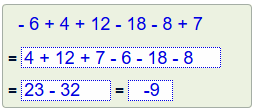Order the positive and negative numbers Find the sum of the positive numbers Find the sum of the negative numbers Get the total resultClick on  new  to create a new problem.

Can you top 295 points?

#### Add and subtract integerswith strategy -profi-

Note the calculation strategy.
 = = =

realmath.de

... more than just practicing